# Characteristic Vibration of Air-Column(Open Tube)

In the previous lecture, we discussed characteristic vibrations using stringed instruments as an example.
Let us consider wind instruments in the same way.

## Why do wind instruments sound?

In the case of stringed instruments, the strings vibrate to produce sound.
So what is vibrating in a wind instrument?
It is not the instrument itself, is it?

Picture the shape of a wind instrument.
All wind instruments are cylindrical and filled with air.

In wind instruments, the air inside the instrument is vibrating!

The air inside the cylinder is called the air-column.
With wind instruments, it is not the structure of the instrument that should be considered, but the air-column within it.

Now let us see how the air-column vibrates.

## What are the characteristic vibrations of an air-column?

Let us consider the recorder as a representative of wind instruments.
A simple model of the recorder, ignoring the fine shapes and holes, is shown in the figure below.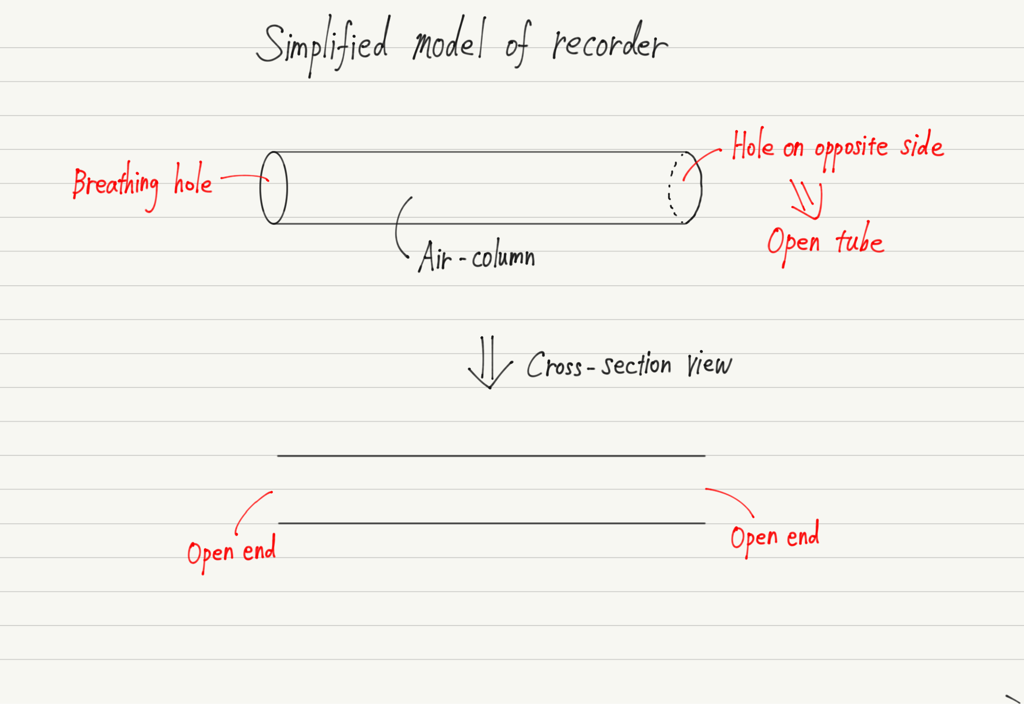Such a tube that is open on both sides is called an open tube.
The recorder is blown in from one side, causing the air inside to vibrate.

Now, there is an important point in dealing with air-column vibrations.
That is, the vibration does not go out of the tube.

Since the open tube has openings on both sides, it would seem that vibrations coming from the left side would pass directly to the right.
In reality, however, it is pushed back in at the exit of the tube!

This is caused by the pressure difference between inside and outside the tube.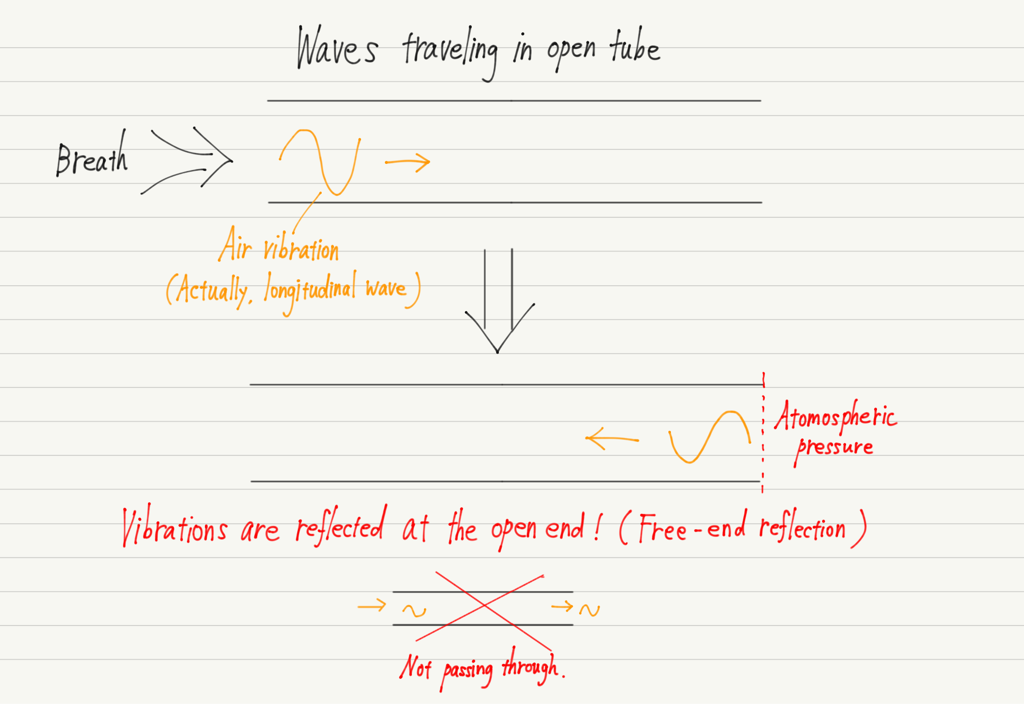As a result, the waves traveling rightward in the tube (incident wave) and the waves being pushed back (reflected wave) overlap, creating a stationary wave!

Now you can see the connection to the previous lecture.
Whether it is a string or wind instrument, the underlying principle is the stationary wave.

By the way, a stationary wave generated in a string is node at both ends (because the string is fixed at both ends).
In the case of an open tube, the air is free to vibrate at the open end, which is the loop of the stationary wave.

## Types of characteristic vibrations in open tube

Now, let’s draw a picture and discuss the characteristic vibration in an open tube.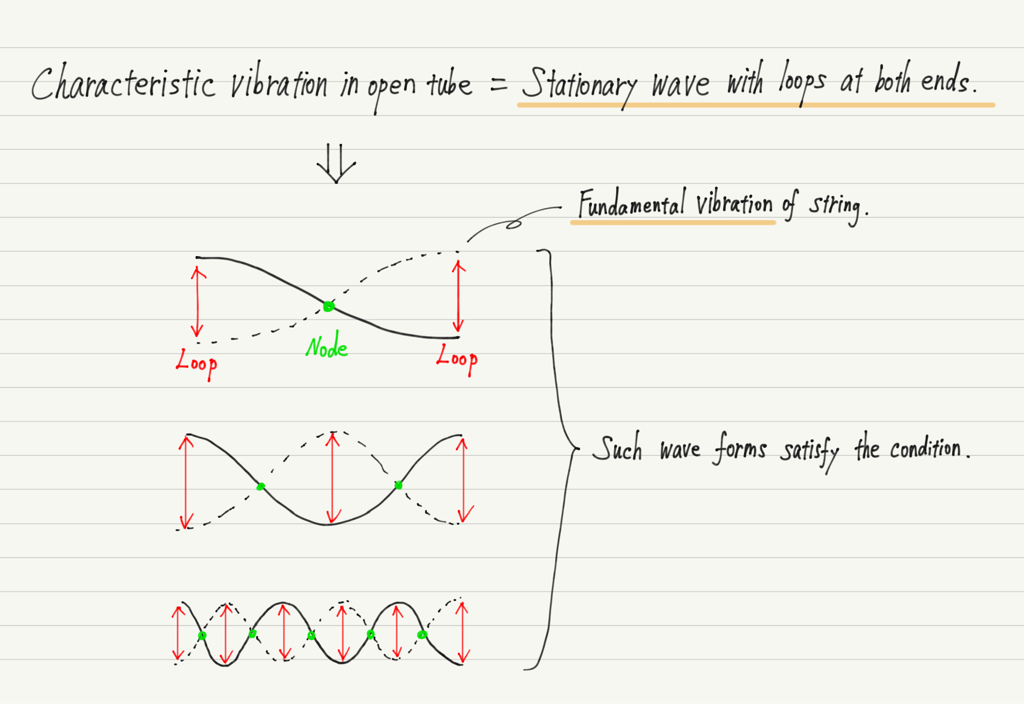As with the string, there are several possible stationary waves, but the simplest (least number of loops and nodes) is called the fundamental vibration.
And, as with the string, the vibration of the form of the fundamental vibration with n attached to it is called n-th harmonic vibration.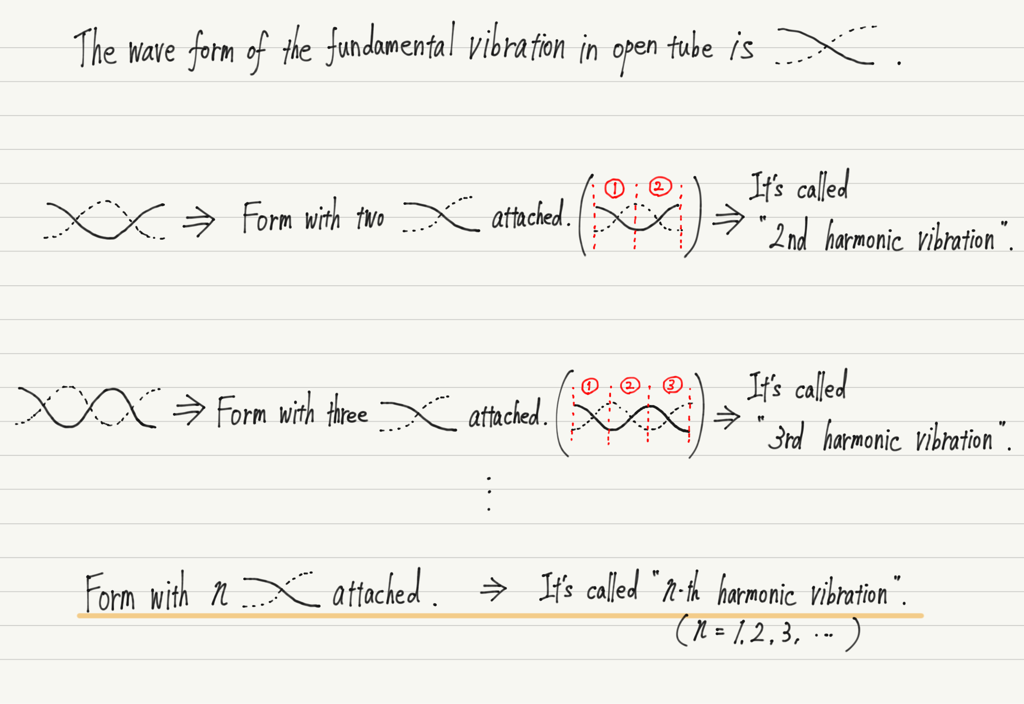Inside a wind instrument, these vibrations are mixed together to produce the unique sound of the instrument.

## Consideration of characteristic vibrations in open tube

Based on the shape of the stationary wave, let’s find the eigen frequencies, just as we did with the string!

Let us consider fundamental, 2nd harmonic, and 3rd harmonic vibration with the length of the air-column as L.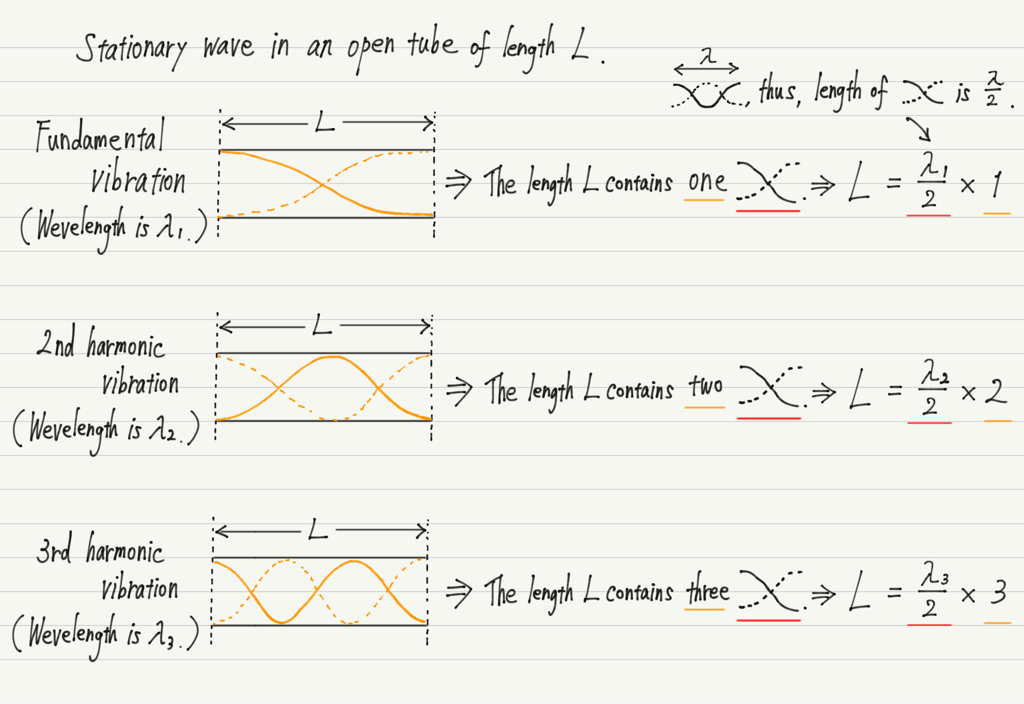Same procedure as for the string, except that both ends are loop and the shape of the fundamental vibration has changed!

All that remains is to use v=fλ.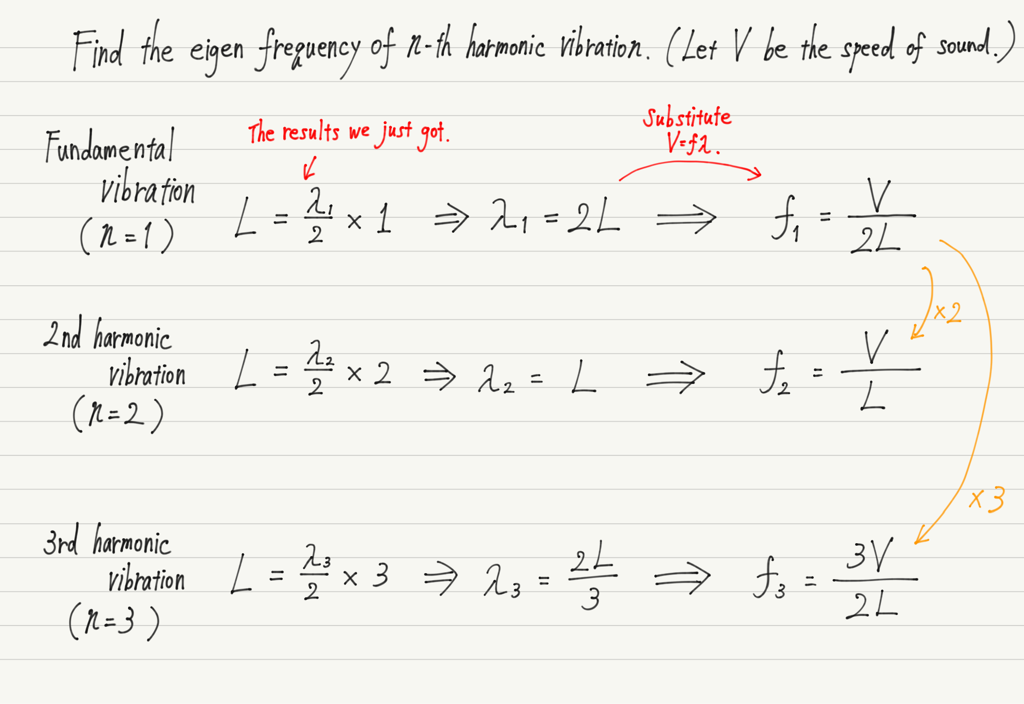The characteristic vibration of the string and the form of the equation look exactly alike, but note the speed of the waves in the equation!
In the case of an air-column, V represents the speed of waves traveling through the air.
That is, the speed of sound!
(The v in the equation for the characteristic vibration of the string is not the speed of sound, but the speed at which the string’s vibration is transmitted.)

Let’s find it for n-th harmonic vibration (n = 1, 2, 3, …) too!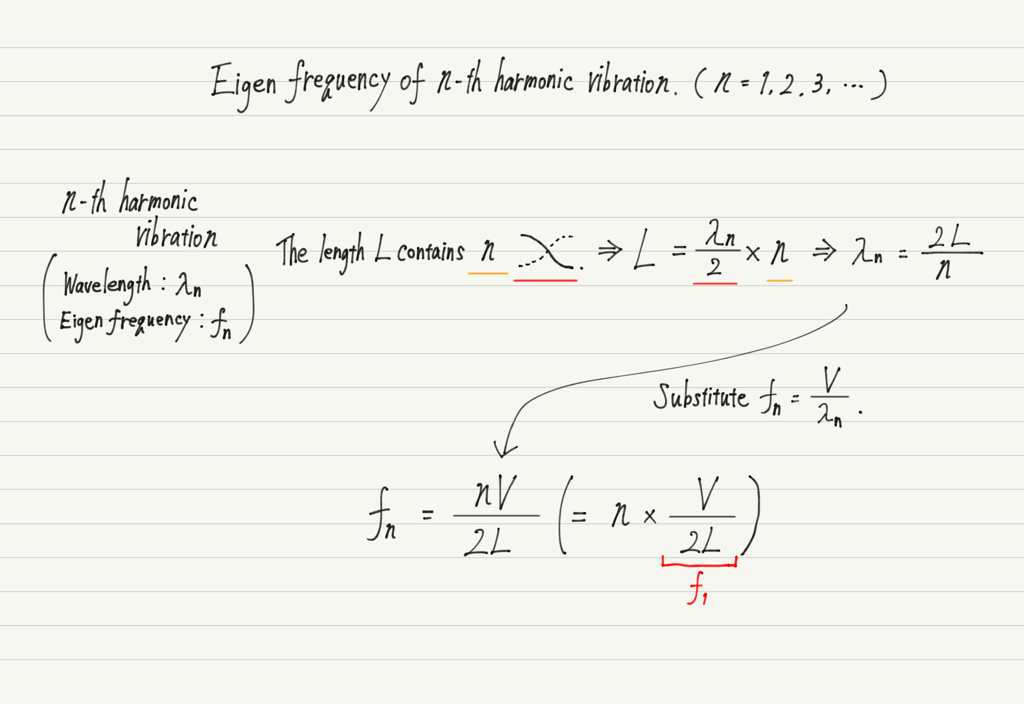As with the string, we see that the eigen frequency of the n-th harmonic vibration is n times the fundamental vibration.

## Wind instruments and Eigen frequency

In the case of a string, the eigen frequency was determined by the length of the string and the speed of wave propagation.

In the case of a tube, the speed of wave propagation is constant at the speed of sound, so the eigen frequency is determined only by the length of the air-column.

From the equation obtained earlier, we see that the eigen frequency is inversely proportional to the length of the air-column.
The longer the air column, the smaller the frequency.
That’s why the lower-pitched instruments are so huge!

By the way, the trombone changes its pitch by changing the length of the tube by moving it in and out.
How do instruments other than the trombone change scales?

Recorders and other wind instruments do not change length while playing, but they do change pitch by opening or closing holes in their bodies.
If the hole is left open, the waves will reflect at that point, so only the length of the air-column can be varied without changing the length of the instrument itself.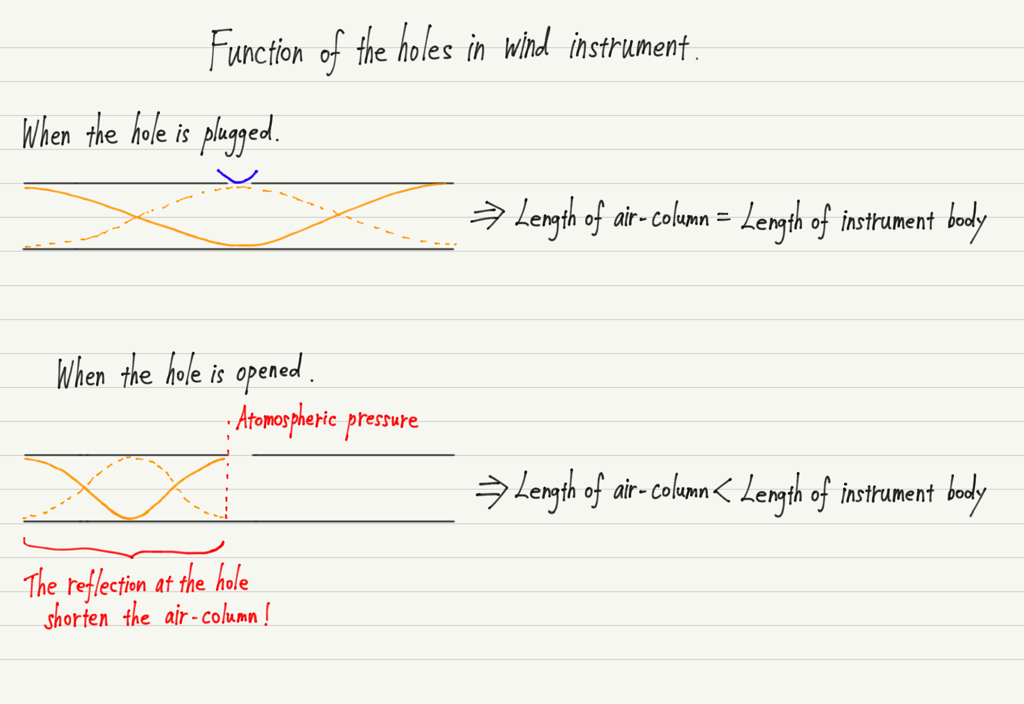If you plug all the holes, you will use the full length of the instrument, which is the lowest note it can produce!

## Summary of this lecture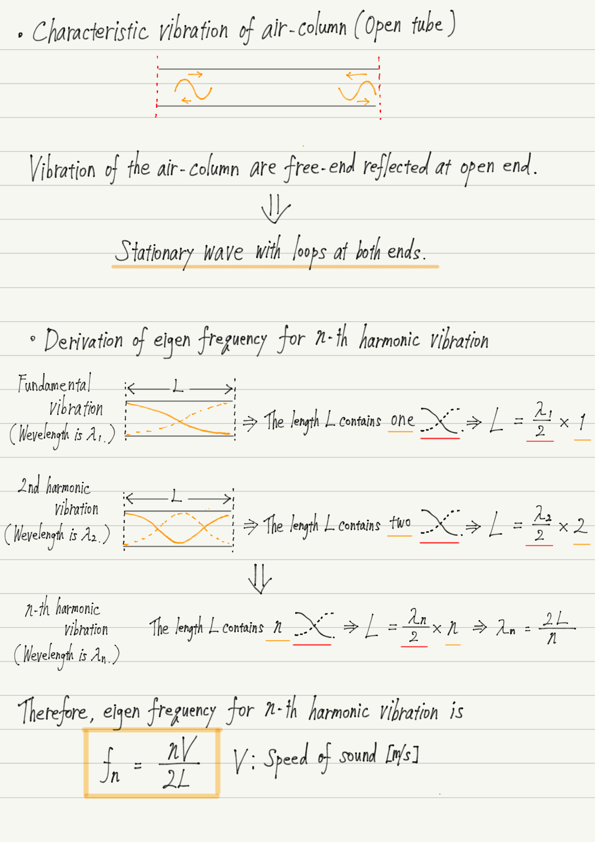## Next Time

Next time we’ll look at tubes open on only one side!

https://www.yukimura-physics.com/entry/wave-f20

error: Content is protected !!